# RD Sharma Solutions For Class 12 Maths Exercise 19.5 Chapter 19 Indefinite Integrals

RD Sharma Solutions for Class 12 Maths Exercise 19.5 Chapter 19 Indefinite Integrals is provided here. This exercise deals with evaluation of integrals of the form

$$\begin{array}{l}\int (ax+b)\sqrt{cx+d} dx\end{array}$$
and
$$\begin{array}{l}\int \frac{ax+b}{\sqrt{cx+d}} \ dx\end{array}$$
. Experts at BYJU’S have formulated the RD Sharma Class 12 Solutions for Maths in the most lucid and easy manner.

Solutions are developed using shortcut techniques to help students grasp the concepts faster and to make learning fun. The solutions to this exercise are available in the pdf format, which can be downloaded easily from the links provided below. To clear their doubts students can refer to RD Sharma Solutions for Class 12 Maths Chapter 19 Exercise 19.5.

## Download the PDF of RD Sharma Solutions For Class 12 Chapter 19 – Indefinite Integrals Exercise 19.5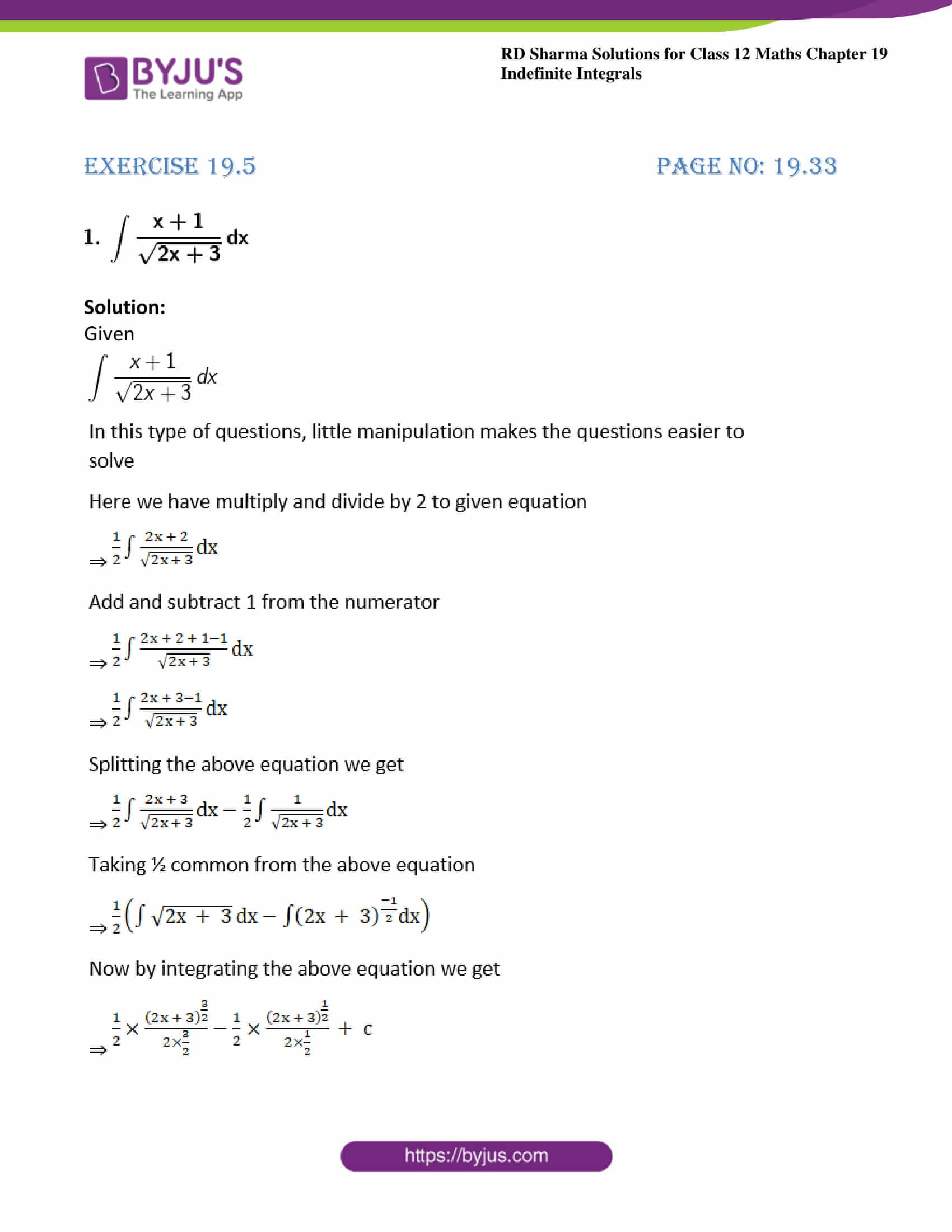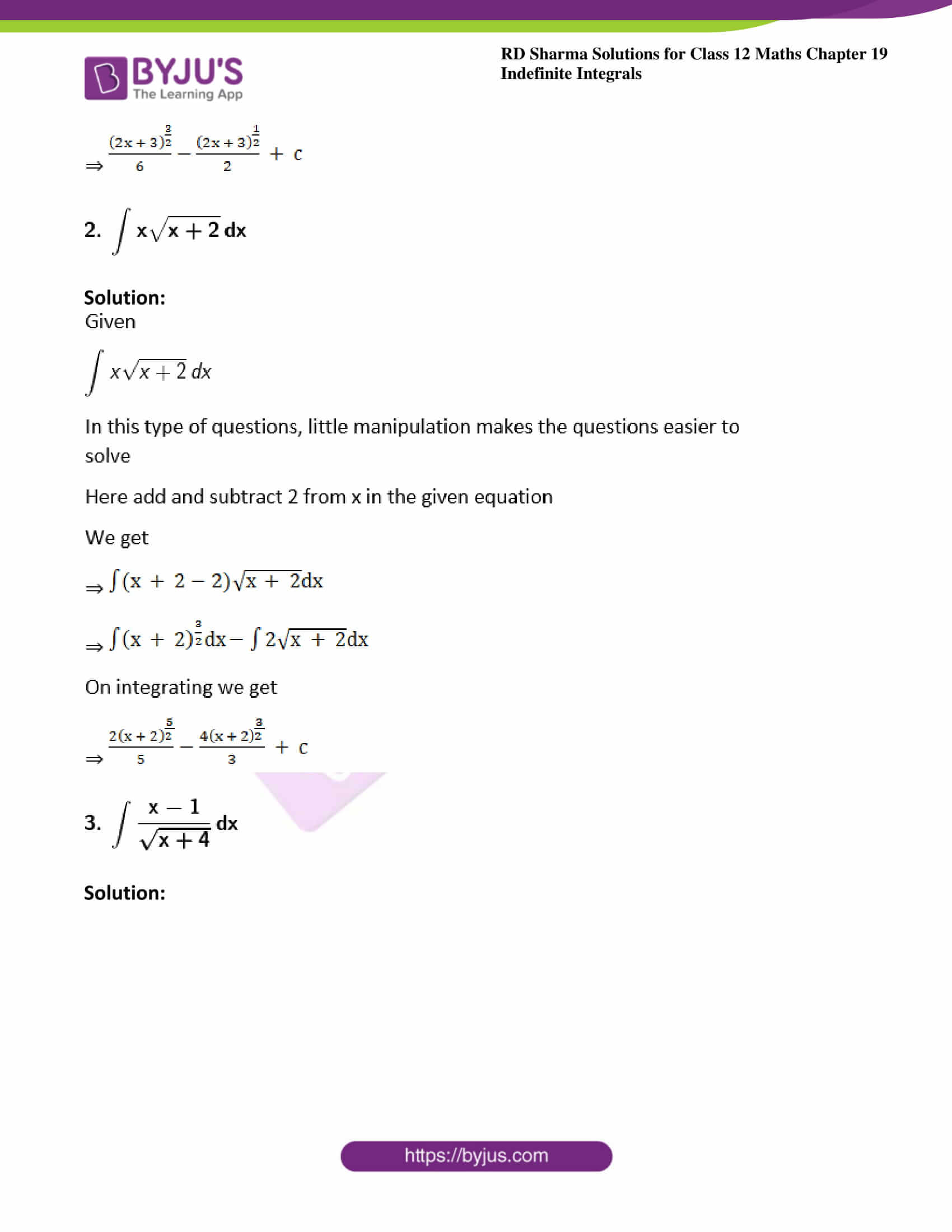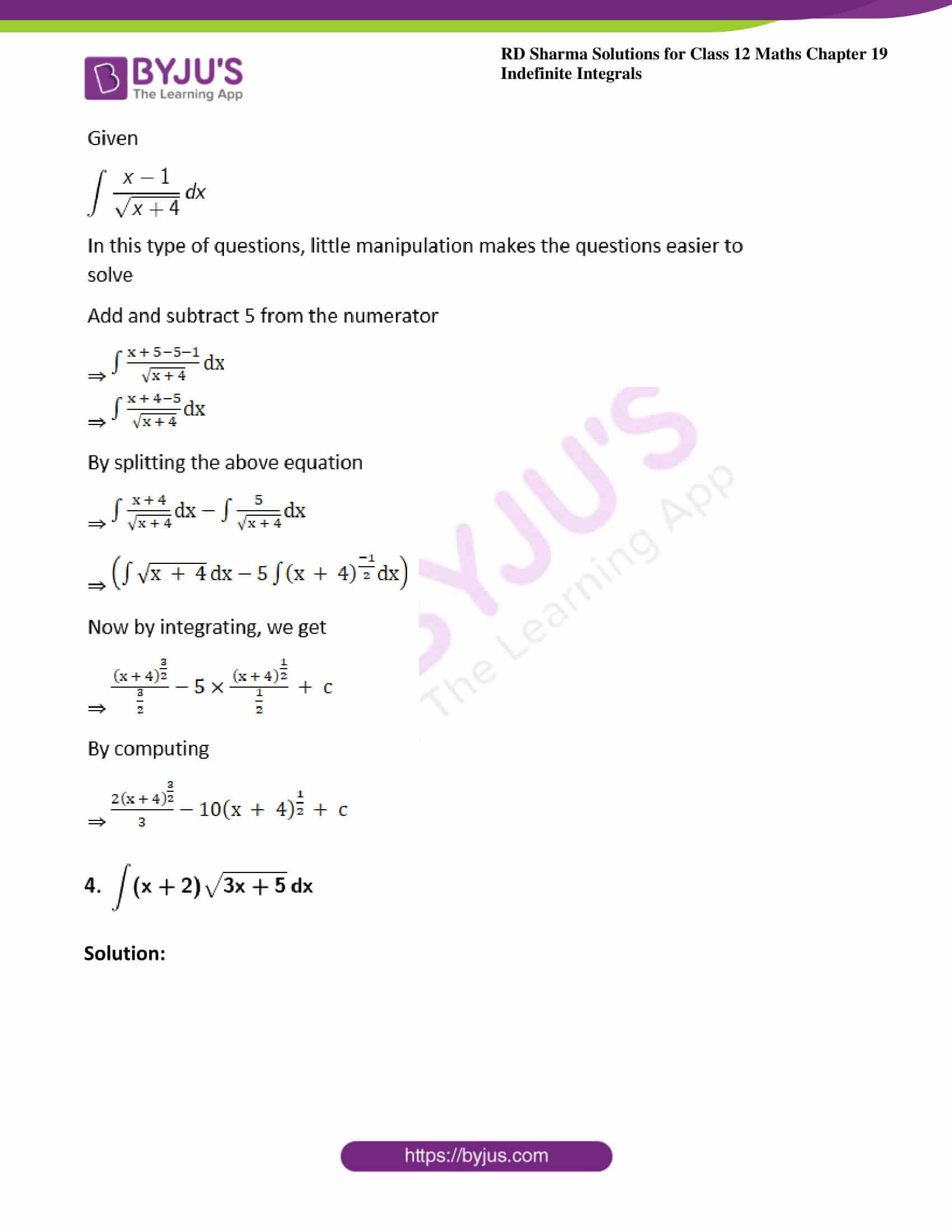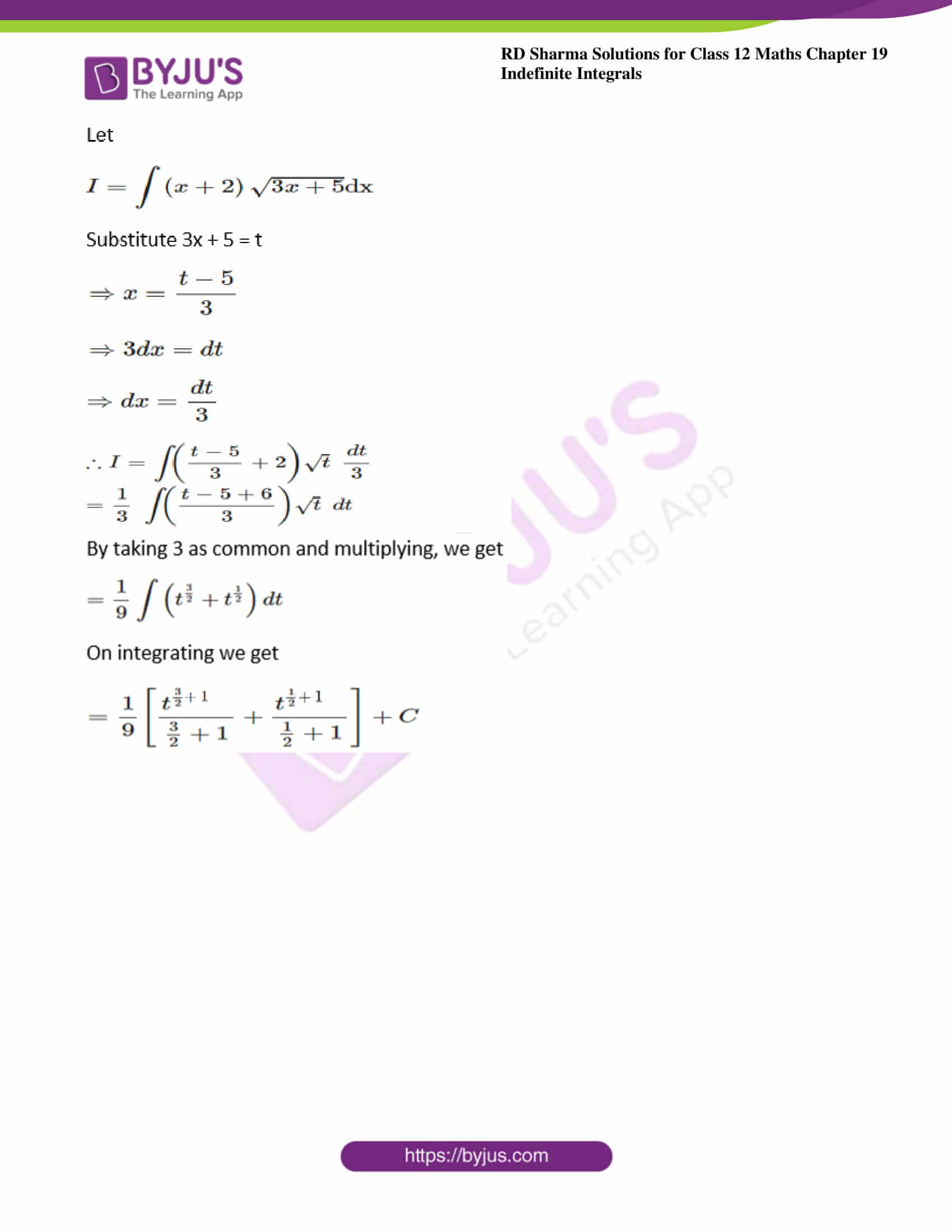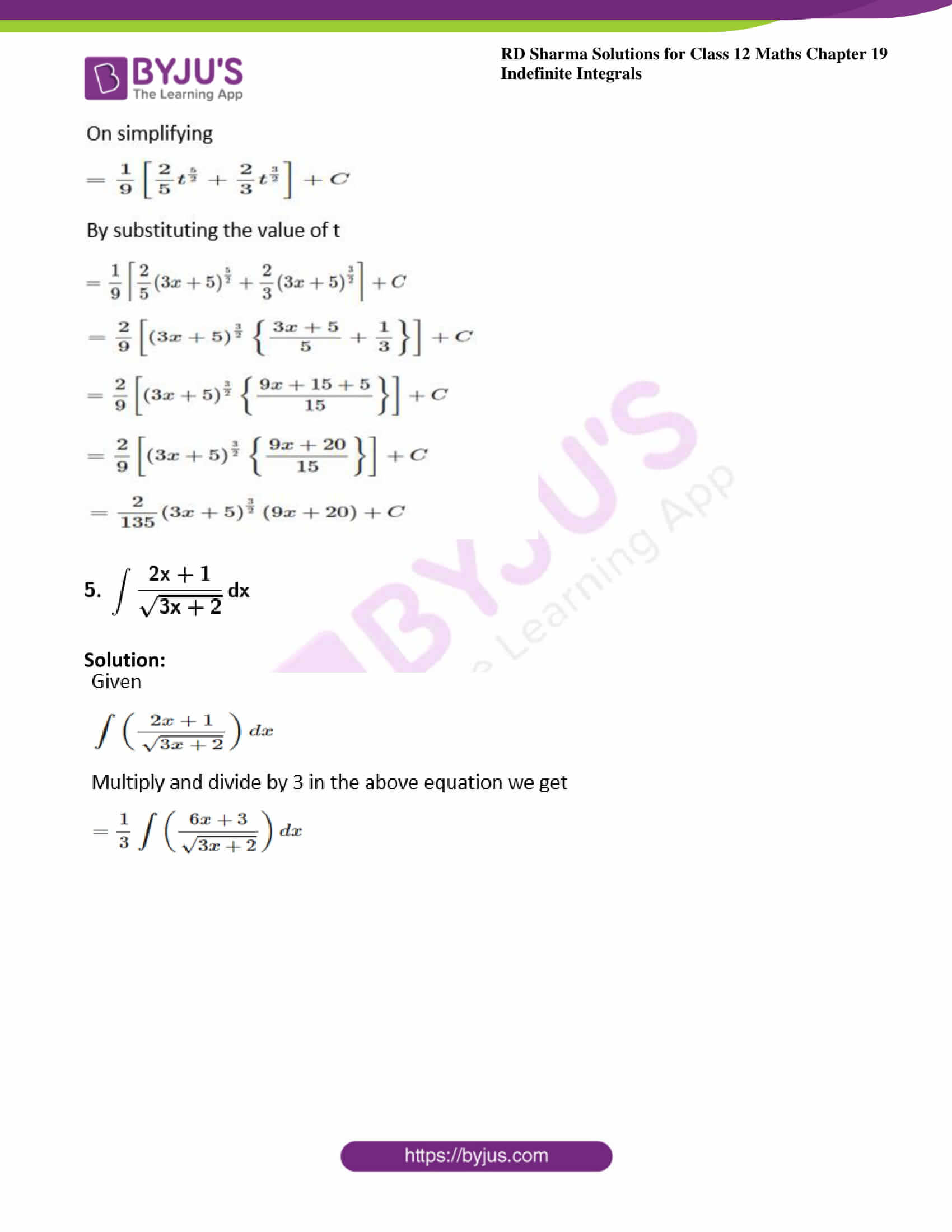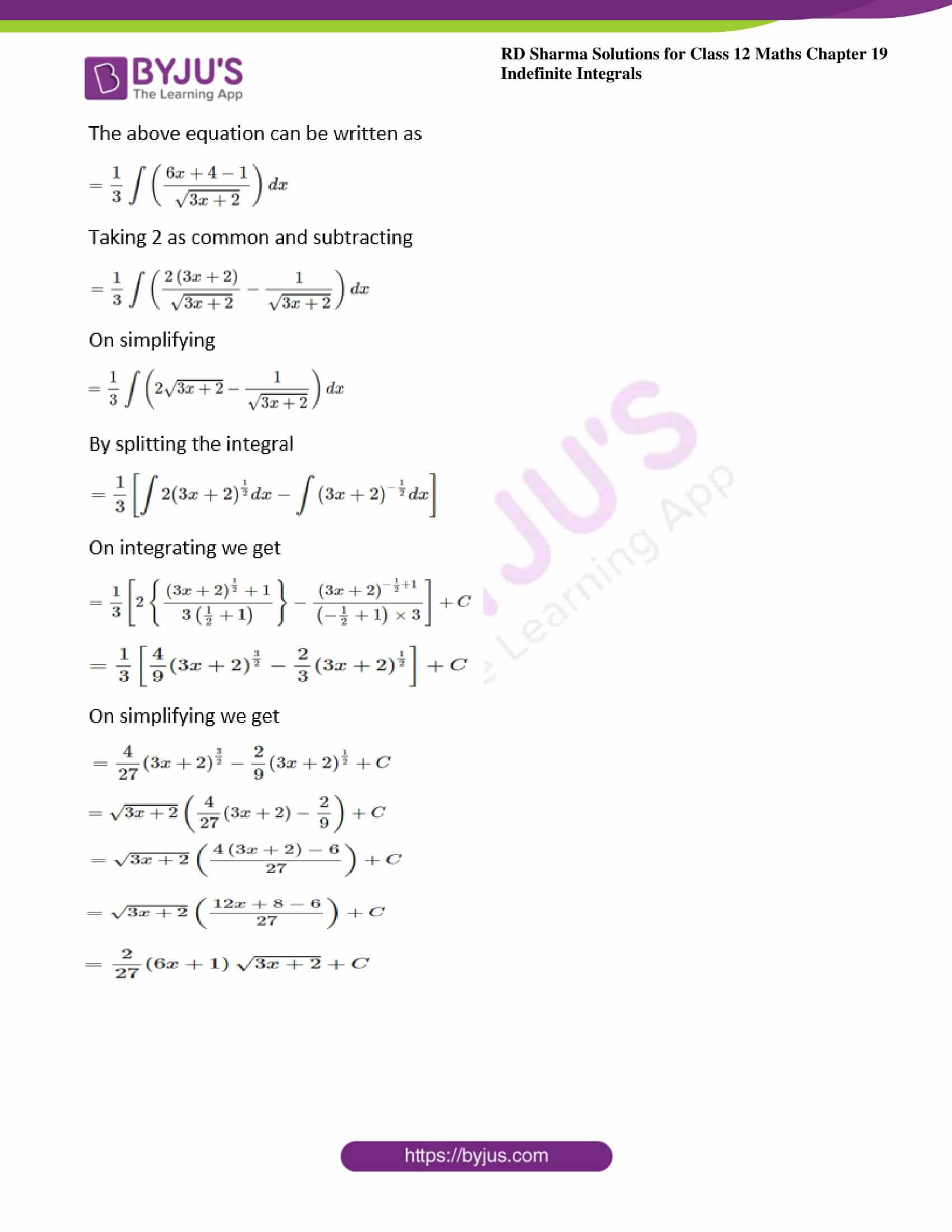### Exercise 19.5 Page No: 19.33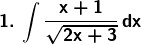Solution:

Given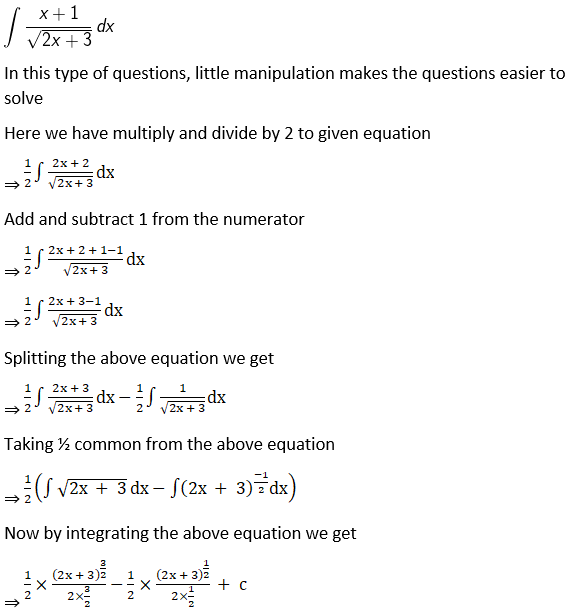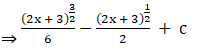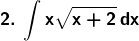Solution: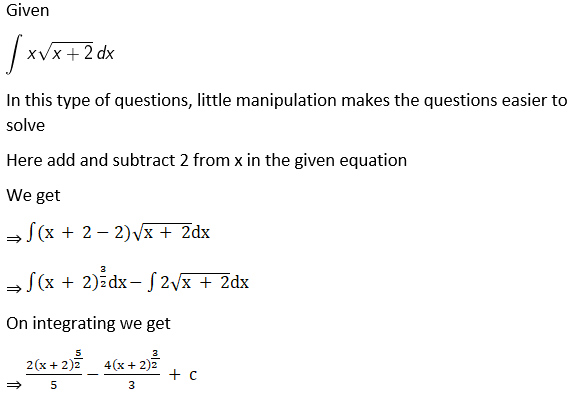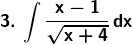Solution: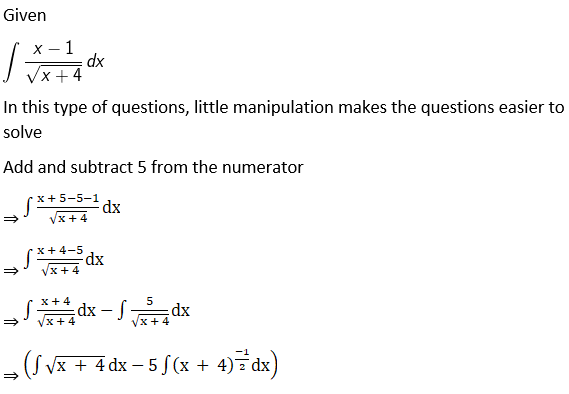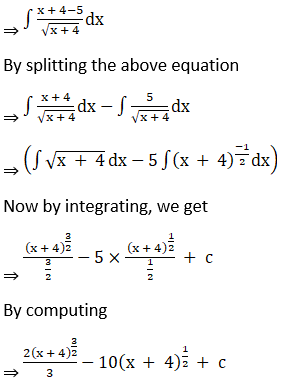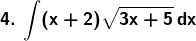Solution: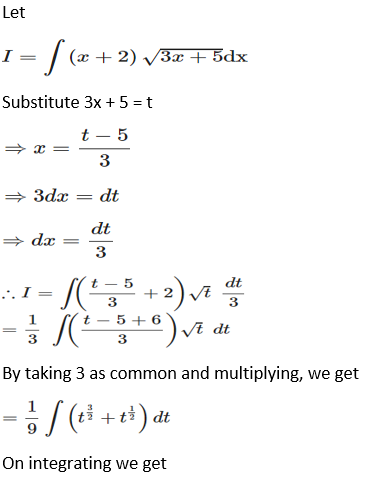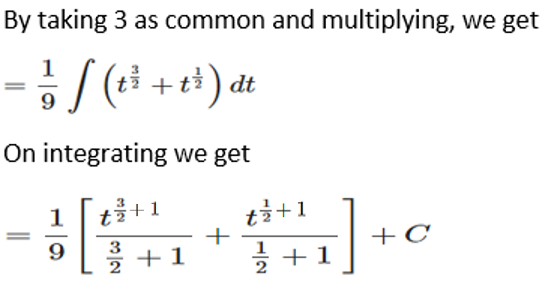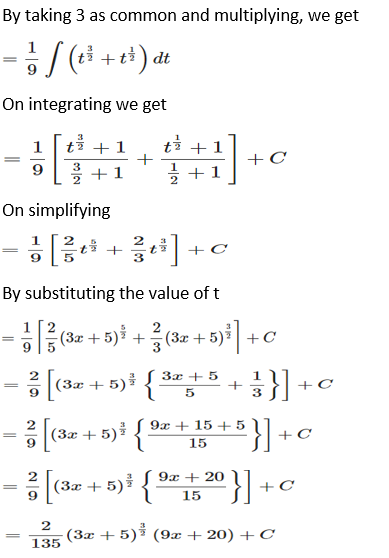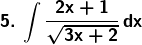Solution: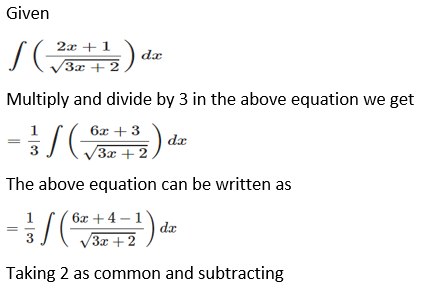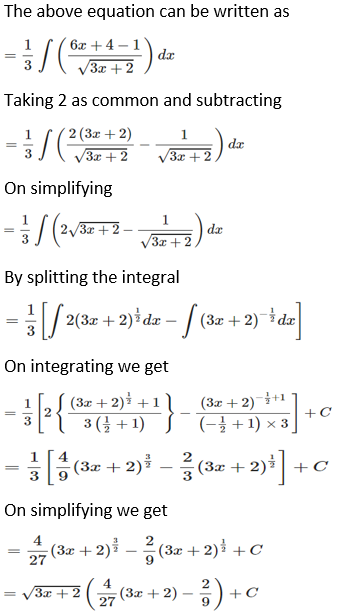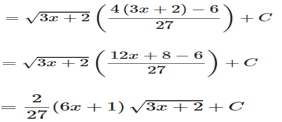### Access other exercises of RD Sharma Solutions For Class 12 Chapter 19 – Indefinite Integrals

Exercise 19.1 Solutions

Exercise 19.2 Solutions

Exercise 19.3 Solutions

Exercise 19.4 Solutions

Exercise 19.6 Solutions

Exercise 19.7 Solutions

Exercise 19.8 Solutions

Exercise 19.9 Solutions

Exercise 19.10 Solutions

Exercise 19.11 Solutions

Exercise 19.12 Solutions

Exercise 19.13 Solutions

Exercise 19.14 Solutions

Exercise 19.15 Solutions

Exercise 19.16 Solutions

Exercise 19.17 Solutions

Exercise 19.18 Solutions

Exercise 19.19 Solutions

Exercise 19.20 Solutions

Exercise 19.21 Solutions

Exercise 19.22 Solutions

Exercise 19.23 Solutions

Exercise 19.24 Solutions

Exercise 19.25 Solutions

Exercise 19.26 Solutions

Exercise 19.27 Solutions

Exercise 19.28 Solutions

Exercise 19.29 Solutions

Exercise 19.30 Solutions

Exercise 19.31 Solutions

Exercise 19.32 Solutions